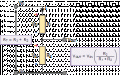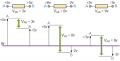# Demanding task in the field of electromagnetism

#### akhu

Joined Jan 3, 2021
39

''A potentiometer with R = 100 Ohm is connected to an operating voltage of Ub = 10 V. At the center tap, 6V are measured against ground. At time t = 15 ms, an inductance to ground with L = 1 H is connected to the center tap. ''

2.1: Calculate the current Iv that the voltage source must deliver at time t = 0ms.

2.2: At time t = 40ms, calculate the current in the coil IL, its voltage UL and the current that the voltage source must supply.

2.3: Calculate for t-> infinite the current Iv that the voltage source has to deliver.

2.5 After a long time (t-> infinite) the voltage source is suddenly separated from the potentiometer. What happens to the inductance, what voltage is on the coil (calculation!) And how does it work?

My questions:

1.) The center tap probably means the center tap of a trimming potentiometer, right?

2.) Is it always about a real voltage source when talking about an operating voltage? Depending on that, I would have to draw another equivalent circuit diagram.

3.) Does it have an impact on my circuit if the technical current has to flow through my coil first before it goes through? a resistance can flow? So what would happen if I swapped coil and resistor in the circuit in a series connection?

Moderator edit: Moved to Homework Help

#### SamR

Joined Mar 19, 2019
4,197
What is the proper name for the center connection of a potentiometer?

•akhu

#### akhu

Joined Jan 3, 2021
39
I found a picture on the internet maybe this can help to explain.My first idea was a voltage divider. So I use this circuit diagramm for my calculations.My solutions for:

2.1: Iv = 0.25 A
2.1 UL(0,04s)= 2.01 V and IL(0,04)=0.199 A and the current that the source must supply to that time Iv=50.25 mA
2.3: Iv= 0
2.5 The process ist self indcution.

#### MrChips

Joined Oct 2, 2009
26,121
How did you arrive at
2.1: Iv = 0.25 A

I believe that is not correct.

•akhu

#### akhu

Joined Jan 3, 2021
39
I also dont believe that Iv=0.25 A is not correct. It should be Iv=0.1 A. But then I cant understand the sense of the information that 6V is messured against the ground at the time t=0ms.

But how I'm arrive Iv=0.25 A:

First I calculated R1, that must be R1=40 Ohm. And then Iv= Ub/R1=10V/40 Ohm = Iv = 0.25 A. Because the current which is on R1, muss be the same one that the source supplies.

But please correct me If I'm wrong.

Last edited:

#### SamR

Joined Mar 19, 2019
4,197
Read what the problem says and sketch it out piece by piece. Start with R = 100 Ohm.

•akhu

#### MrChips

Joined Oct 2, 2009
26,121
Your voltage divider is R1 + R2 = 100Ω.

What is the value of R1?
What is the value of R2?

•akhu

#### akhu

Joined Jan 3, 2021
39
R1 = 40 Ohm, and R2 = 60 Ohm. Here you can see

#### MrChips

Joined Oct 2, 2009
26,121
Your last line is not correct.

•akhu

#### akhu

Joined Jan 3, 2021
39
Okay but why ? I mean you If you read, the text above.... They messured 6 V against the ground. Otherwise, can you draw it ? Maybe then I can understand what I did wrong.

#### MrChips

Joined Oct 2, 2009
26,121
What is the voltage across R1?
What is the voltage across R2?
What is the voltage across R1 + R2?

#### akhu

Joined Jan 3, 2021
39
R1= 10V
R2= 10V
R1+R2 = 10VBut so Iv must be 0.1 A right ?

#### MrChips

Joined Oct 2, 2009
26,121
R1= 10V
R2= 10V
R1+R2 = 10VBut so Iv must be 0.1 A right ?
Bizarre.

How can 10V + 10V = 10V?

Voltage is always a measure of potential difference.
VAB = VA - VB

where VA and VB are voltage measurements with respect to some common reference point.